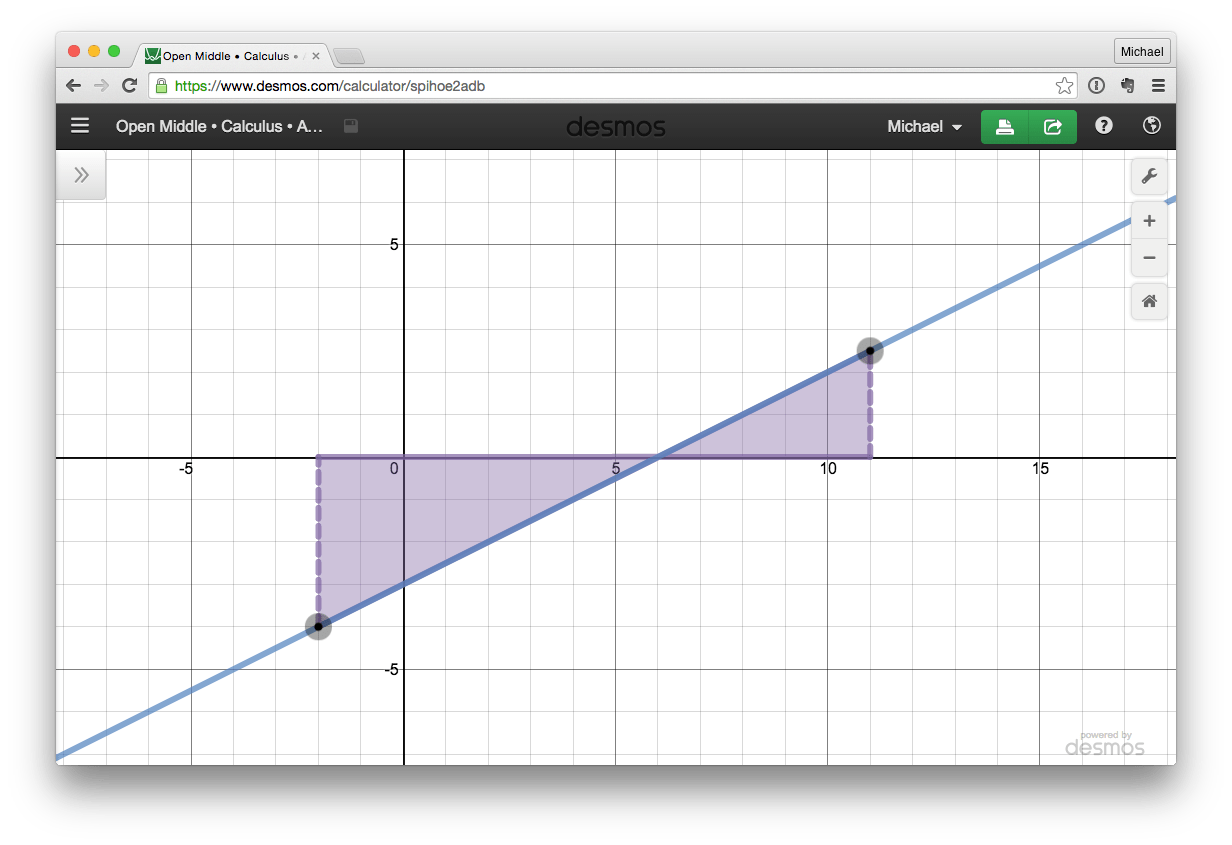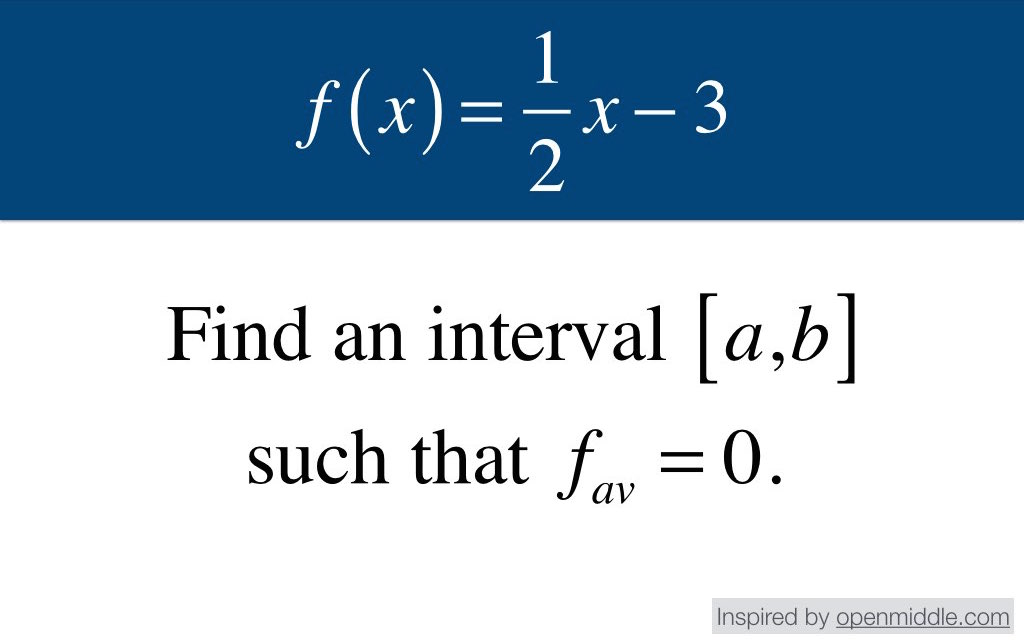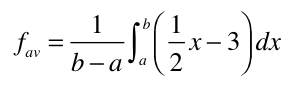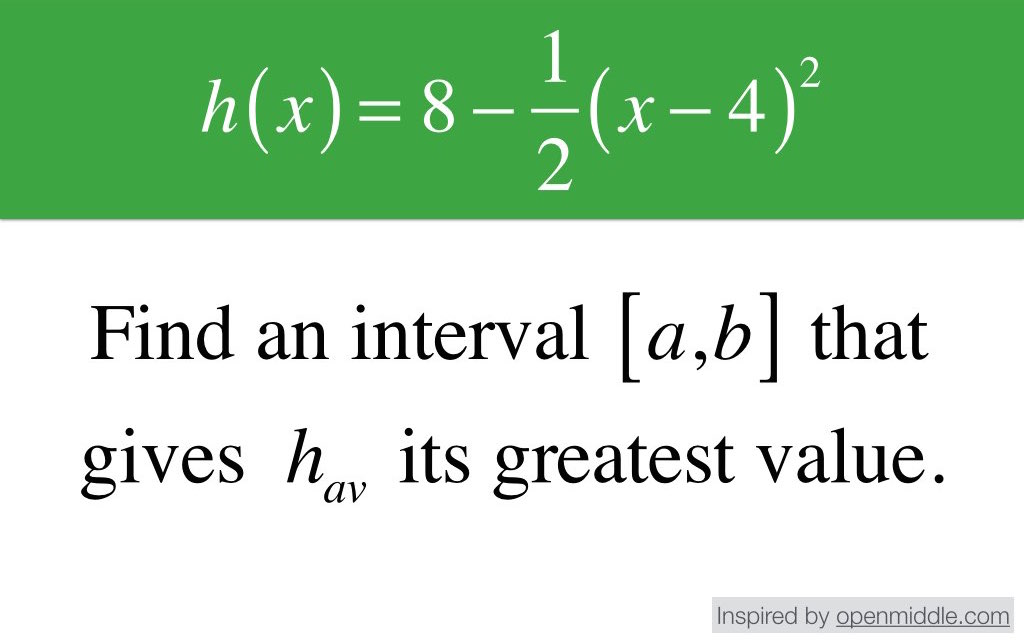# Average Value

Develop student understanding of average value of a function with this series of open-middle calculus challenges:### Challenge

1. Find an interval [ab] on which the average value of the function f(x) is zero.

### Lesson Notes

Display the following image:Prior to presenting the problem to your students, attempt to solve it in as many ways as you can. Here are two possible approaches:

#### Algebraically

Consider the function:The average value is given by:Setting the average value equal to zero—and simplifying—we get:#### Graphically

In order for the average value to equal zero, the integral from a to b must be equal to zero. Therefore…Here are a few examples:

•$[5, 7]$
•$[4, 8]$
•$[3, 9]$
•$[0, 12]$
•$[-2, 14]$

While the algebraic approach requires students to apply and strengthen important skills (integration, algebraic manipulation, looking for and making use of structure), I think the graphical approach offers the greatest opportunity to development conceptual understanding of average value.

Some students may employ a wild guess-and-check approach. That’s not necessarily a bad way to begin, especially if they have access to a calculator with numerical integration built in. However, after some tinkering, encourage them to try one of the above methods (algebraic or graphical).

If students lean heavily on the algebraic approach, invite them to consider the problem graphically as well—especially to make sense of the generalized answer,$a+b=12$.

### Extensions

For students who find a solution to the challenge ahead of classmates, push them further with the following:

1. Find several other intervals. Describe any patterns you see. Generalize your results if possible.
2. Find an interval where the average value is equal to a. (Bonus: Minimize, or maximize, a.)
3. Find an interval where the average value is equal to b. (Bonus: Maximize, or minimize, b.)

### New Functions

You can extend the life of this challenge—either on the same day, or several weeks later for a bit of rich-task-spiral-review—by introducing more complex functions:### Standards

• AB.3.B
Applications of integrals: Average value of a function

### More Lessons

Looking for more lessons? Click here.